Proposed Algorithm for Setting SVT Chip Thresholds

# Proposed Algorithm for Setting SVT Chip Thresholds

Revised: 1/25/99

## Introduction:

The threshold for each SVT front-end chip, which has 128 channels, can be set independently. We would like to set the threshold as low as possible, while maintaining reasonably low noise occupancy, in order to achieve maximum efficiency. Three factors play a role in setting the threshold for a given chip. They are:
• Threshold DAC offset: This is average threshold DAC offset for all of the channels on the chip.
• Noise: Noisy chips will need higher thresholds in order to achieve reasonable noise occupancy levels.
• Threshold DAC offset dispersion: This is the channel-to-channel spread in the threshold DAC offset for the chip. The channels with the highest offsets will limit the chip threshold (excluding bad channels).
The original plan was to determine the threshold DAC offset for each chip by extrapolating the 50% efficiency point to zero injected charge . We now know that the chip-to-chip spread in the calibration DAC offset is much more significant than the spread in the threshold DAC offset in the original method . Experience with the commissioning module  has shown that, to first order, one can assume that the threshold DAC offset is zero.

Assuming the threshold DAC offset is zero, one could use a prescription such as

Threshold = Noise * N1 + Threshold DAC dispersion * N2

where the noise and threshold dispersion are measured from a threshold scan using charge injection and N1 and N2 are chosen appropriately (e.g. N1 = N2 = 3). A complication with this method is that the measured noise depends on the amount of charge injected during the threshold scan. It isn't clear what the noise is for zero injected charge. Pickup from the command lines, which produces early hits, can give artificially high values for the threshold DAC dispersion, which also complicates things. A final complication is that for a few chips the threshold DAC offset may not be zero, as we assume.

We propose that the prescription for setting the thresholds should be to set the threshold such that we get a well defined noise occupancy. Note that it is likely that the desired noise occupancy will be different on different layers ( e.g. the machine background hit rate is a strong function of layer. One can imagine having a higher noise hit rate in the inner layers, as long as it remains small or comparable to the machine background rate.) The easiest way to find the threshold for a given noise hit rate would be to scan the threshold with no charge injection and measure (global) occupancy for a given chip as a function of threshold. This is straightforward but not robust against unmasked bad (noisy) channels. Provided that the DAQ can handle the rate, the desired occupancy should be the occupancy for "good" channels. A procedure to accomplish this goal is outlined below.

## New Method:

The following is a summary of the steps involved in the new method.
1. Do a threshold scan, without the cal_strobe, with Nmax events per threshold. The trigger jitter window is set to its maximum value (31 counts) in order to save time. The noise occupancy can be scaled by the ratio of the data-taking jitter window and the maximum window offline.
2. For each channel, determine the threshold DAC value for which the occupancy (Nhits/Nmax) is Oave. We will refer to this threshold value as Thr(Oave,i) for channel i. This is done by fitting the occupancy as a function of threshold.
3. Make a histogram of the Thr(Oave,i) values for each chip and fit it to a Gaussian (mean M and sigma S).
4. Choose the threshold that gives an average occupancy of Oave for the good channels. The good channels are identified by having Thr(Oave,i) in the range of M-N*S < Thr(Oave,i) < M+N*S where N is 3.5 for example.
The following sections describe these steps in more detail.

## Step 1: Threshold scan

The only question involved with this step is what value of Nmax (number of events per threshold) is sufficient to measure the channel occupancy accurately near an occupancy of Oave. Here, we are limited by how much time we have to do the threshold scan. If we have unlimited time, a fit to the occupancy vs threshold distribution would not be necessary, since we could measure it accurately enough directly by taking a large number of events at every threshold.

## Step 2: Fitting occupancy vs threshold for each channel

To determine the threshold for which the occupancy is at the 1 % level or less, a fit is probably required. The plots below show two threshold scans for a particular channel where the data were taken with no cal-strobe command. The plots on the left (right) are for 100 (10,000) events per threshold.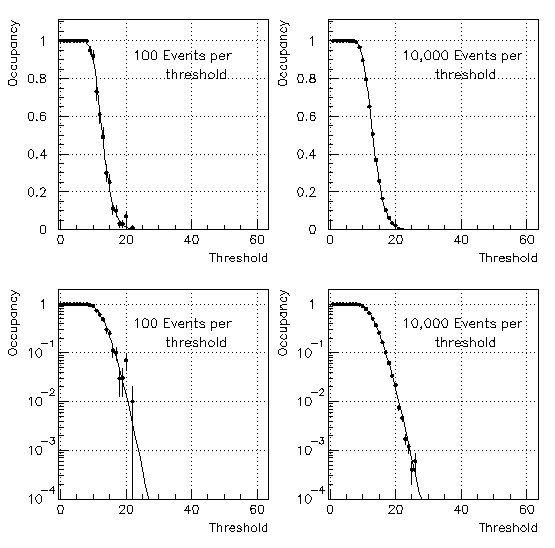The occupancy as a function of threshold is fit to the functional form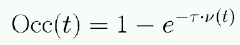whereand

•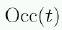is the occupancy at threshold t.
•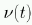is the frequency of noise hits at threshold t.
•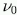is the frequency of noise hits at zero threshold.
•is the jitter time.
•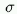is the noise (free to float in the fit).
•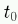is the threshold offset (free to float in the fit).
The interpretation of the parameters in the fit as they are labled above may not be valid, but what matters is that the function can be used to fit the data well. The fit can done using the Newton-Raphson method  in a closed form. By "closed" we mean there are no unlimited iterations or dependencies on other software packages, such as MINUIT.

## Step 3: Fitting the histogram of Thr(Oave,i) values

The top plot below shows the histogram of threshold values (Thr(Oave,i)) for which the occupancy is 1.0% for a given chip. The lower plot shows Thr(Oave,i) as a function of channel number for this chip.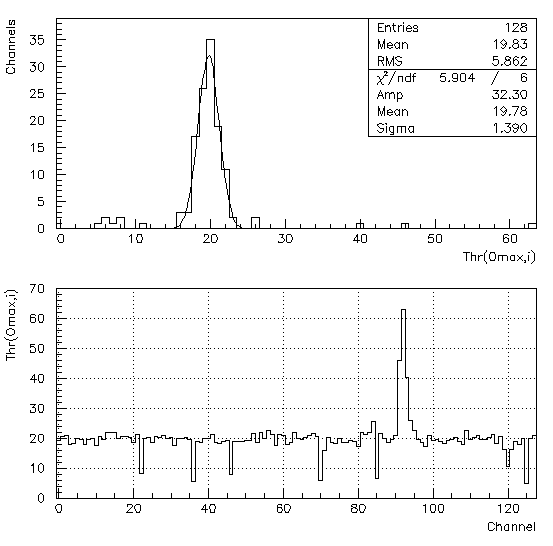There are a few unbonded channels which have Thr(Oave,i) values at or below 10 dac counts. There is also a group of noisy channels near channel number 93. Fitting the top histogram is an effective way to determine the average behavior of the good channels without being influenced by bad (noisy and unbonded) channels.

## Step 4: Choosing the threshold for data taking

In order to get a sensible threshod, it is essential to identify bad channels (noisy or unbonded) and remove them from the calculation of the occupancy for the chip. For example, if one channel is stuck on and it is not removed from the average occupancy for the chip, the average occupancy will be at least 1/128 = 0.78% no matter what threshold you choose.

We propose only using channels which have

M-N*S < Thr(Oave,i) < M+N*S,

where N is 3.5 for example, for calculating the occupancy of the chip. This effectively removes unbonded and noisy channels from the calculation of the occupancy. The occupancy for each good channel is given by the fit described in Step 2. The set threshold is the one that gives an average occupancy of Oave for the good channels.

## The algorithm applied to layers 1 and 2 after installation

We have applied the algorithm described above to data taken with layers 1 and 2 after installation on the cones. The data are from run 20206 which used a shaping time of 100 ns. The jitter window was set to it's maximum value (31) and the scan was done without sending the cal_strobe command. All of the plots below will show results for three target occupancies: 0.1%, 2.0%, and 5.0%.

The histograms below show the spectrum of set thresholdsThe three plots below show the chip average occupancy for the three different target occupancies. The solid histogram uses only the good channels ( M-N*S < Thr(Oave,i) < M+N*S, ) for the calculation. The dashed histogram uses all nominally used channels.Note that while a few bad chanels may not strongly affect the average occupancy once the threshold is selected, they can make selecting the threshold very difficult. Also note that the width of the distribution of chip occupancies scales with the target occupancy. This is because the slope of the occupancy vs threshold curve increases with occupancy, so a one count difference is a larger difference in occupancy for larger occupancies.

The plots below show the spectrum of channel occupancies.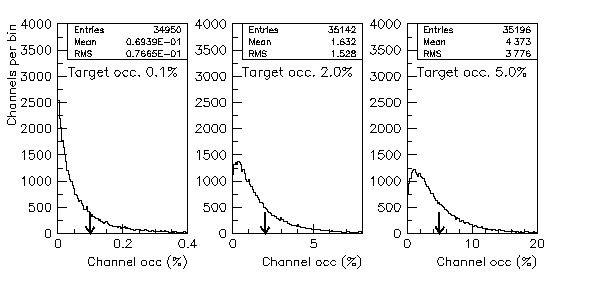## References:

1) SVT Gains and Offsets from UCSB TestBench. (go back to text)

5) Louis Lyons, Statistics for nuclear and particle physics,
Cambridge University Press (1986) p. 134-137. (go back to text)

Revised: 1/25/99
owen@electron.physics.ucsb.edu
claudio@muon.physics.ucsb.edu